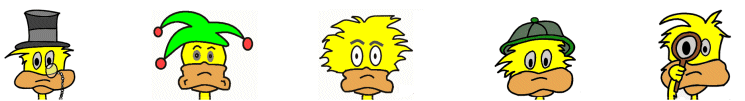Math >> Money and Finance

# Money Math

## Word Problems with Multiplication and Division

Below are money word problems that use multiplication and division. The student will also need to understand the units of United States currency including coins (dimes, nickels, etc.), cents, and dollars.

1) Joey makes \$250 each month. How much money will he make in a year?

\$250 x 12 = \$3000

He will make \$3000 in a year.

2) Sophia wants to buy 14 oranges. The oranges cost 35 cents each. How much money will 14 oranges cost?

14 x 35 = 490 cents or \$4.90

3) If you purchased 24 pencils for \$5.28. How much did each pencil cost?

\$5.28 ÷ 24 = \$0.22 or 22 cents each

4) Anna is trying to save her money for a new bike. Each week she puts \$5.50 in savings. The bike she wants to buy costs \$88. How many weeks will it take her to save enough money to buy the bike?

88 ÷ 5.50 = 16

It will take her 16 weeks to save enough money.

5) Benjamin went to the clothes store and purchased a blue shirt for \$18.45, a red shirt for \$22.18, a green shirt for \$28.97. What was the average price he paid for a shirt?

To figure the average we must total the cost of the shirts and the divide by the total number of shirts (which is three).

\$18.45 + \$22.18 + \$28.97 = \$69.60 total cost

\$69.60 ÷ 3 = \$23.20.

The average price that Benjamin paid for a shirt was \$23.20.

6) A gallon of gas costs \$2.90. How much would it cost to fill up a car with a 15 gallon tank?

\$2.90 x 15 = \$43.50

7) At the store you see two sizes of peanut butter jars. One size is 23.96 ounces and sells for \$5.99. The other size is 12.5 ounces and sells for \$3.00. Which is the better deal per ounce?

Figure out the per ounce price of the first jar:

\$5.99 ÷ 23.96 ounces = .25 or 25 cents per ounce

Now, figure out the per ounce price of the other jar:

\$3.00 ÷ 12.5 ounces = .24 or 24 cents per ounce

The second jar is a better deal because it is less money per ounce.

8) The bill at a restaurant is \$38.00. The service was good, so you decide to tip 20%. What is the new total with tip included?

First figure out the tip:

\$38.00 x .2 = \$7.60

Another way to figure this out is to move the decimal over one place and double the amount. This would be \$3.80 x 2 which is \$7.60.

Next, add the tip to the bill:

\$38.00 + \$7.60 = \$45.60

9) Alice purchased 18 bolts of cloth. Each bolt has 12 yards of cloth. A yard of cloth costs \$2.35. How much money did all the cloth cost Alice?

First figure out how much each bolt of cloth cost:

12 yards times \$2.35 for each yard = 12 x \$2.35 = \$28.20 per bolt

Now multiply the bolt cost by the number of bolts purchased:

18 bolts x \$28.20 = \$507.60

Alice spent \$507.60 total on cloth.

10) Three friends made \$435 together each month for a year cleaning houses. At the end of the year they divided the money evenly. How much money did each friend earn for the year?

First figure out how much money they made together in a year:

\$435 x 12 = \$5220

Divide the total up between the three friends:

\$5220 ÷ 3 = \$1740

Note: This information is not to be used for individual legal, tax, or investment advice. You should always contact a professional financial or tax advisor before making financial decisions.
Math >> Money and Finance